Test: Multiplication And Division - 3

# Test: Multiplication And Division - 3

Test Description

## 20 Questions MCQ Test Mathematics for Class 4: NCERT | Test: Multiplication And Division - 3

Test: Multiplication And Division - 3 for Class 4 2023 is part of Mathematics for Class 4: NCERT preparation. The Test: Multiplication And Division - 3 questions and answers have been prepared according to the Class 4 exam syllabus.The Test: Multiplication And Division - 3 MCQs are made for Class 4 2023 Exam. Find important definitions, questions, notes, meanings, examples, exercises, MCQs and online tests for Test: Multiplication And Division - 3 below.
Solutions of Test: Multiplication And Division - 3 questions in English are available as part of our Mathematics for Class 4: NCERT for Class 4 & Test: Multiplication And Division - 3 solutions in Hindi for Mathematics for Class 4: NCERT course. Download more important topics, notes, lectures and mock test series for Class 4 Exam by signing up for free. Attempt Test: Multiplication And Division - 3 | 20 questions in 40 minutes | Mock test for Class 4 preparation | Free important questions MCQ to study Mathematics for Class 4: NCERT for Class 4 Exam | Download free PDF with solutions
 1 Crore+ students have signed up on EduRev. Have you?
Test: Multiplication And Division - 3 - Question 1

### Find the missing term in the following.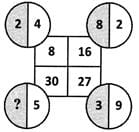Detailed Solution for Test: Multiplication And Division - 3 - Question 1

2 × 4  = 8, 5 × 2  = 10, 9 × 3 = 27 Similarly, 5 × 6 = 30.

Test: Multiplication And Division - 3 - Question 2

### If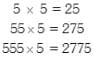find the product of 55555×5

Detailed Solution for Test: Multiplication And Division - 3 - Question 2

55555 × 5 = 277775

Test: Multiplication And Division - 3 - Question 3

### Direction: Based on the following table answer the following questions.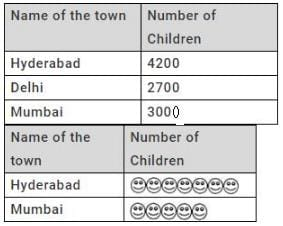Q. How many children does each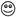represent?

Detailed Solution for Test: Multiplication And Division - 3 - Question 3

Hyderabad = 4200 = 7 × 600 Mumbai = 3000 =5 × 600 So, each  represents 600 children.

Test: Multiplication And Division - 3 - Question 4

Direction: Based on the following table answer the following questions.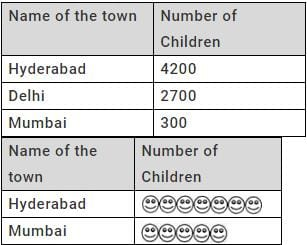Q. Which of the following is the correct representation of the number of children in Delhi?

Detailed Solution for Test: Multiplication And Division - 3 - Question 4

Delhi = 2700 = 4 × 600 + 300  So number of children in Delhi can be represented as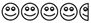Test: Multiplication And Division - 3 - Question 5

What is the other way of writing 205 × 6?

Detailed Solution for Test: Multiplication And Division - 3 - Question 5

(200 + 5) × 6 = 200 × 6 + 5 × 6

Test: Multiplication And Division - 3 - Question 6

Direction: Based on the following figures and the given table shows which the number of squares formed by the number of dots.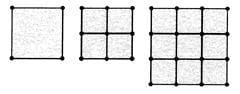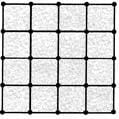? and so on.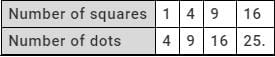Q. How many dots are required to form 25 squares?

Detailed Solution for Test: Multiplication And Division - 3 - Question 6

If the number of squares is 1 = 1 × 1 the  number of dots required = 2 × 2 = 4. If the number of squares is 4 = 2 × 2 the number of dots required = 3 × 3 = 9. If the number of squares is 9 = 3 × 3 The number of dots required = 4 × 4 = 16 If the number of squares is 16 the number of dots required = 5 × 5 = 25. So, to form 25 squares =5 × 5, the number of dots required = 6 × 6 = 36.

Test: Multiplication And Division - 3 - Question 7

Direction: Based on the following figures and the given table shows which the number of squares formed by the number of dots.? and so on.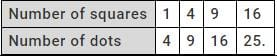Q. How many squares are formed by 49 dots?

Detailed Solution for Test: Multiplication And Division - 3 - Question 7

Number of squares formed by 49 dots = 6 × 6 = 36

Test: Multiplication And Division - 3 - Question 8

Observe the following multiplications and find the product 707×707.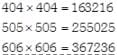Detailed Solution for Test: Multiplication And Division - 3 - Question 8

Observation is, 404 × 404 = 163216 = (4 × 4) (16 + 16) (4 × 4) 505 × 505 = 255025 = (5 × 5) (25 + 25) (5 × 5) 606 × 606 = 367236 = (6 × 6) (36 + 36) (6 × 6) So, 707 × 707 =(7 × 7)(49 + 49)(7 × 7) = 499849

Test: Multiplication And Division - 3 - Question 9

Identify the product 15 × 31 × 78 estimated by rounding each number to the nearest ten.

Test: Multiplication And Division - 3 - Question 10

What is the product of the place values of "5" as shown on the spike abacus?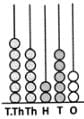Test: Multiplication And Division - 3 - Question 11

How many minutes were there in the month of February, 2004?

Test: Multiplication And Division - 3 - Question 12

Pandu and Raghu wrote two 2-digit numbers, each having the digit 7 only once and in the place shown.
Pandu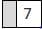& Raghu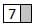If Pandu wrote the greatest two digit number and Raghu wrote the least two digit number, what is their product?

Detailed Solution for Test: Multiplication And Division - 3 - Question 12

The greatest two digit number written by Pandu is 97. The least two digit number written by Raghu is 70. Their product = 97 × 70 = 6790

Test: Multiplication And Division - 3 - Question 13

Find the product of the numbers shown on the spike abacuses.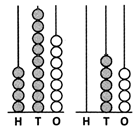Detailed Solution for Test: Multiplication And Division - 3 - Question 13

497 × 54 = 26838

Test: Multiplication And Division - 3 - Question 14

A factory manufactures 1617 shoes in a week. How many shoes does it manufacture in a day?

Detailed Solution for Test: Multiplication And Division - 3 - Question 14

1 week = 7 days
∴ No. of shoes manufactured in a day = 231

Test: Multiplication And Division - 3 - Question 15

The product of two numbers is 476. If one number is 34, what is the other number?

Detailed Solution for Test: Multiplication And Division - 3 - Question 15

The other number = 476 ÷ 34 = 14

Test: Multiplication And Division - 3 - Question 16

If 5 transistors cost Rs. 1525, find the cost of each transistor.

Detailed Solution for Test: Multiplication And Division - 3 - Question 16

The cost of each transistor  = 1525 ÷ 15 = 305

Test: Multiplication And Division - 3 - Question 17

Madhu divided 3250 by 18 and obtained a quotient of 179 and remainder of 28.Forthisproblem, which of these checks will help Madhu to find out his mistakes?

Detailed Solution for Test: Multiplication And Division - 3 - Question 17

He has to check if Dividend = Quotient × Divisor + Remainder.

Test: Multiplication And Division - 3 - Question 18

Study the given number sentences.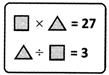If these number sentences are true, which of the following may be correct?

Detailed Solution for Test: Multiplication And Division - 3 - Question 18

If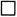= 3 and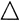= 9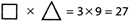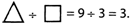Test: Multiplication And Division - 3 - Question 19

The exact value of a dollar compared to the Indian rupee changes from day to day. On a given day, Rs. 288 was worth 6 dollars. How many dollars would Rs. 480 have been worth on that day?

Detailed Solution for Test: Multiplication And Division - 3 - Question 19

The value of 1 dollar = 288 ÷ 6 = Rs. 48
∴ The required number of dollars = 480 ÷ 48 = 10

Test: Multiplication And Division - 3 - Question 20

Murali arranged some marbles as shown.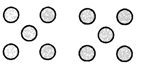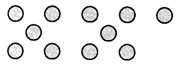Which of the following division facts is best represented by grouping of marbles?
(All the marbles must be considered)

Detailed Solution for Test: Multiplication And Division - 3 - Question 20

The arrangement of marbles clearly indicates 21 ÷ 5 gives quotient 4 and remainder 1.

## Mathematics for Class 4: NCERT

24 videos|44 docs|32 tests
 Use Code STAYHOME200 and get INR 200 additional OFF Use Coupon Code
Information about Test: Multiplication And Division - 3 Page
In this test you can find the Exam questions for Test: Multiplication And Division - 3 solved & explained in the simplest way possible. Besides giving Questions and answers for Test: Multiplication And Division - 3, EduRev gives you an ample number of Online tests for practice

## Mathematics for Class 4: NCERT

24 videos|44 docs|32 tests